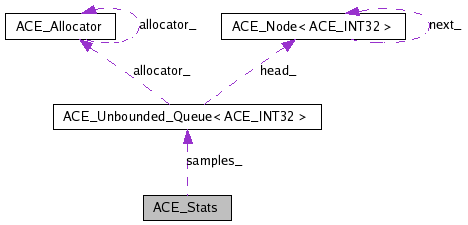# ACE_Stats Class Reference

Provides simple statistical analysis. More...

`#include <Stats.h>`

Collaboration diagram for ACE_Stats:[legend]

List of all members.

## Public Member Functions

ACE_Stats (void)
Default constructor.
int sample (const ACE_INT32 value)
ACE_UINT32 samples (void) const
Access the number of samples provided so far.
ACE_INT32 min_value (void) const
Value of the minimum sample provided so far.
ACE_INT32 max_value (void) const
Value of the maximum sample provided so far.
void mean (ACE_Stats_Value &mean, const ACE_UINT32 scale_factor=1)
int std_dev (ACE_Stats_Value &std_dev, const ACE_UINT32 scale_factor=1)
int print_summary (const u_int precision, const ACE_UINT32 scale_factor=1, FILE *=stdout) const
void reset (void)
Initialize internal state.
void dump (void) const
Print summary statistics to stdout.

## Static Public Member Functions

static void quotient (const ACE_UINT64 dividend, const ACE_UINT32 divisor, ACE_Stats_Value &quotient)
Utility division function, for ACE_UINT64 dividend.
static void quotient (const ACE_Stats_Value &dividend, const ACE_UINT32 divisor, ACE_Stats_Value &quotient)
Utility division function, for ACE_Stats_Value dividend.
static void square_root (const ACE_UINT64 n, ACE_Stats_Value &square_root)

## Protected Attributes

u_int overflow_
ACE_UINT32 number_of_samples_
Number of samples.
ACE_INT32 min_
Minimum sample value.
ACE_INT32 max_
Maximum sample value.
ACE_Unbounded_Queue< ACE_INT32 > samples_
The samples.

## Detailed Description

Provides simple statistical analysis.

Simple statistical analysis package. Prominent features are:

1. It does not use any floating point arithmetic.
2. It handles positive and/or negative sample values. The sample value type is ACE_INT32.
3. It uses 64 bit unsigned, but not 64 bit signed, quantities internally.
4. It checks for overflow of internal state.
5. It has no static variables of other than built-in types.

Example usage:

``` * ACE_Stats stats;
* for (u_int i = 0; i < n; ++i)
* {
* const ACE_UINT32 sample = ...;
* stats.sample (sample);
* }
* stats.print_summary (3);
* ```

## Constructor & Destructor Documentation

 ACE_INLINE ACE_Stats::ACE_Stats ( void )

Default constructor.

## Member Function Documentation

 int ACE_Stats::sample ( const ACE_INT32 value )

Provide a new sample. Returns 0 on success, -1 if it fails due to running out of memory, or to rolling over of the sample count.

 ACE_INLINE ACE_UINT32 ACE_Stats::samples ( void ) const

Access the number of samples provided so far.

 ACE_INLINE ACE_INT32 ACE_Stats::min_value ( void ) const

Value of the minimum sample provided so far.

 ACE_INLINE ACE_INT32 ACE_Stats::max_value ( void ) const

Value of the maximum sample provided so far.

 void ACE_Stats::mean ( ACE_Stats_Value & mean, const ACE_UINT32 scale_factor = `1` )

Access the mean of all samples provided so far. The fractional part is to the specified number of digits. E.g., 3 fractional digits specifies that the fractional part is in thousandths.

 int ACE_Stats::std_dev ( ACE_Stats_Value & std_dev, const ACE_UINT32 scale_factor = `1` )

Access the standard deviation, whole and fractional parts. See description of {mean} method for argument descriptions.

 int ACE_Stats::print_summary ( const u_int precision, const ACE_UINT32 scale_factor = `1`, FILE * file = `stdout` ) const

Print summary statistics. If scale_factor is not 1, then the results are divided by it, i.e., each of the samples is scaled down by it. If internal overflow is reached with the specified scale factor, it successively tries to reduce it. Returns -1 if there is overflow even with a 0 scale factor.

 void ACE_Stats::reset ( void )

Initialize internal state.

 void ACE_Stats::quotient ( const ACE_UINT64 dividend, const ACE_UINT32 divisor, ACE_Stats_Value & quotient ) ` [static]`

Utility division function, for ACE_UINT64 dividend.

 void ACE_Stats::quotient ( const ACE_Stats_Value & dividend, const ACE_UINT32 divisor, ACE_Stats_Value & quotient ) ` [static]`

Utility division function, for ACE_Stats_Value dividend.

 void ACE_Stats::square_root ( const ACE_UINT64 n, ACE_Stats_Value & square_root ) ` [static]`

Sqrt function, which uses an oversimplified version of Newton's method. It's not fast, but it doesn't require floating point support.

 ACE_INLINE void ACE_Stats::dump ( void ) const

Print summary statistics to stdout.

## Member Data Documentation

 u_int ACE_Stats::overflow_` [protected]`

Internal indication of whether there has been overflow. Contains the errno corresponding to the cause of overflow.

 ACE_UINT32 ACE_Stats::number_of_samples_` [protected]`

Number of samples.

 ACE_INT32 ACE_Stats::min_` [protected]`

Minimum sample value.

 ACE_INT32 ACE_Stats::max_` [protected]`

Maximum sample value.

 ACE_Unbounded_Queue ACE_Stats::samples_` [protected]`

The samples.

The documentation for this class was generated from the following files:

Generated on Wed Apr 23 02:41:48 2008 for ACE by1.5.5